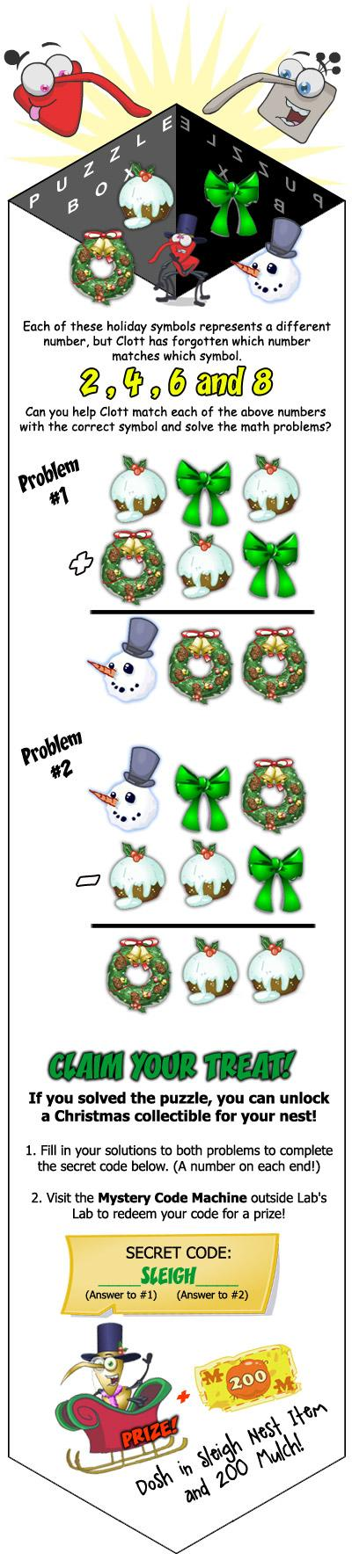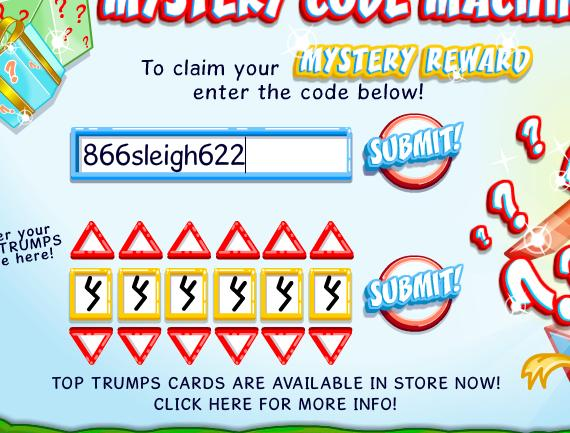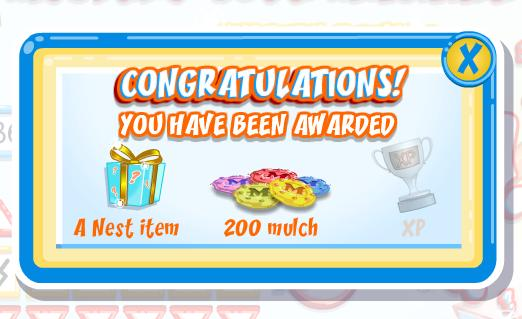# December 11 Weekend Puzzle Challenge Cheats

Clott from Bin Weevils has posted a puzzle challenge on their site. We will provide the solution for the puzzle after the image below.As the above image states, each different symbol stands for either 2, 4, 6, or 8. The answer to problem 1 is 866, and the answer to problem 2 is 622. This makes the final code 866sleigh622.

Let’s look at how I deduced the answer to the two problems:

1. We will use C to represent the cupcake, W to represent the wreath, B to represent the bowtie, and S to represent the snowman.
2. When adding two single digit numbers, the highest number you can carry over is 1. You can verify this by adding the two greatest one digit number, which will give you 18 (you will only be able to carry over a 1).
3. We know you won’t need to carry at all in the above math problem. This is because if you have a remainder of 1, you will have an odd number somewhere in the problem. There aren’t any symbols to represent odd numbers, so we know there aren’t any odd numbers.
4. We know none of the addends can contain the number 8. This is because 8 added to the smallest possible other number (2) would require you to carry. As we mentioned previously in step 3, you will not need to carry in this problem.
5. We can conclude S (the snowman) represents the number 8. This is because that’s the only digit that doesn’t appear in any of the addends (similar to what was mentioned in step 4).
6. We know C + W is 8, based on what we deduced in step 5. We know C or W are either 2 or 6. They cannot be 4 because they are two different numbers that sum up to 8.
7. Because neither C or W is 4 as we mentioned in step 6, we know the remaining B is 4.
8. Looking at the ones column, we know C + 4 = W. As mentioned in step 6, C and W are either 2 or 6. In this case, it makes sense to say C is 2 and W is 6.

After you have the code (866sleigh622), go to Lab’s Lab and click on Mystery’s Nest Items. There, you will enter the above code.You will receive a nest item and 200 mulch!